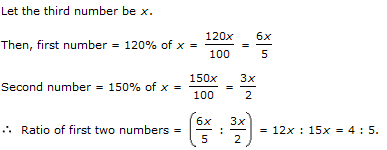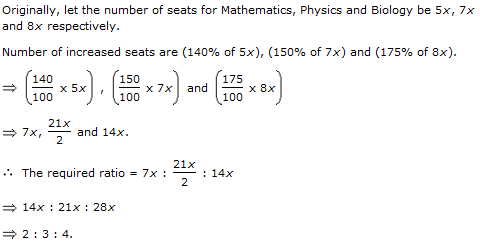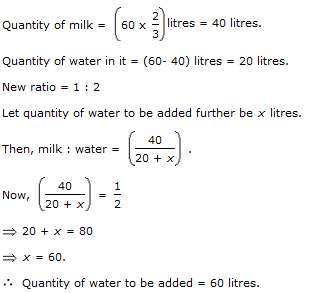1.

A and B together have Sterling 1210. If 4/15 of A's amount is equal to 2/5 of B's amount, how much amount does B have?

 A. Sterling 460 B. Sterling 484 C. Sterling 550 D. Sterling 664
Answer: Option B
Explanation: 4/15A = 2/5B

A = (2/5 x 15/4)B

A = 3/2 B

A/B = 3/2

A : B = 3 : 2.

B's share = Sterling (1210 x 2/5) = Sterling 484.

2.

Two numbers are respectively 20% and 50% more than a third number. The ratio of the two numbers is:

 A. 2 : 5 B. 3 : 5 C. 4 : 5 D. 6 : 7
Answer: Option C
Explanation:3.

A sum of money is to be distributed among A, B, C, D in the proportion of 5 : 2 : 4 : 3. If C gets Euro 1000 more than D, what is B's share?

 A. Euro 500 B. Euro 1500 C. Euro 2000 D. None of these
Answer: Option C
Explanation: Let the shares of A, B, C and D be Euro 5x, Euro 2x, Euro 4x and Euro 3x respectively.

Then, 4x - 3x = 1000

x = 1000.

B's share = Euro 2x = Euro (2 x 1000) = Euro 2000.

4.

Seats for Mathematics, Physics and Biology in a school are in the ratio 5 : 7 : 8. There is a proposal to increase these seats by 40%, 50% and 75% respectively. What will be the ratio of increased seats?

 A. 2 : 3 : 4 B. 6 : 7 : 8 C. 6 : 8 : 9 D. None of these
Answer: Option A
Explanation:5.

In a mixture 60 litres, the ratio of milk and water 2 : 1. If the this ratio is to be 1 : 2, then the quanity of water to be further added is:

 A. 20 litres B. 30 litres C. 40 litres D. 60 litres
Answer: Option D
Explanation: